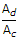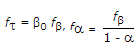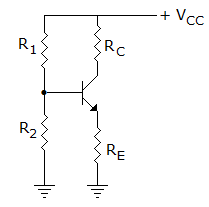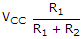# Electronics and Communication Engineering - Analog Electronics

46.

If the differential voltage gain and the common mode voltage gain of a differential amplifier are 48 dB and 2 dB respectively, then its common mode rejection ratio is

 A. 23 dB B. 25 dB C. 46 dB D. 50 dB

Explanation:

We know that CMMR =20 log (CMMR) = 20 log Ad - 20 log Ac = 48 - 246 dB.

47.

An npn transistor has a Beta cutoff frequency fβ of 1 MHz, and a common emitter short circuit low frequency current gain β0 of 200. It unity gain frequency fT and the alpha cut off frequency fa2 respectively are

 A. 200 MHz, 201 MHz B. 200 MHz, 199 MHz C. 199 MHz, 200 MHz D. 201 MHz, 200 MHz

Explanation:.

48.

In figure if the transistor is cut off, the collector voltage is equal toA. VCC B. 0.5 VCC C.D. zero

Explanation:

Since transistor is cut off, IC = 0 and VC = VCC .

49.

If input frequency is 50 Hz, the frequency of output wave in a full wave diode rectifier circuit is

 A. 25 Hz B. 50 Hz C. 100 Hz D. 200 Hz

Explanation:

There are two output waves for one input wave.

50.

A class A transformer coupled power amplifier is to deliver 10 W output. The power rating of transistor should not be less than

 A. 5 W B. 10 W C. 20 W D. 40 W